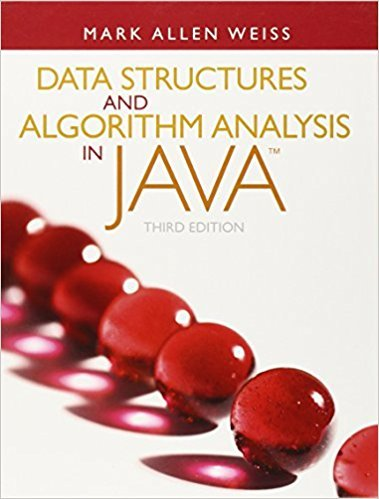×
Get Full Access to Data Structures And Algorithm Analysis In Java - 3 Edition - Chapter 7 - Problem 7.40
Get Full Access to Data Structures And Algorithm Analysis In Java - 3 Edition - Chapter 7 - Problem 7.40

×

# The following divide-and-conquer algorithm is proposed for finding theISBN: 9780132576277 316

## Solution for problem 7.40 Chapter 7

Data Structures and Algorithm Analysis in Java | 3rd Edition

• Textbook Solutions
• 2901 Step-by-step solutions solved by professors and subject experts
• Get 24/7 help from StudySoup virtual teaching assistantsData Structures and Algorithm Analysis in Java | 3rd Edition

4 5 1 298 Reviews
10
5
Problem 7.40

The following divide-and-conquer algorithm is proposed for finding the simultaneousmaximum and minimum: If there is one item, it is the maximum and minimum,and if there are two items, then compare them and in one comparison you can findthe maximum and minimum. Otherwise, split the input into two halves, dividedas evenly as possibly (if N is odd, one of the two halves will have one more elementthan the other). Recursively find the maximum and minimum of each half, andthen in two additional comparisons produce the maximum and minimum for theentire problem.a. Suppose N is a power of 2. What is the exact number of comparisons used bythis algorithm?b. Suppose N is of the form 3 2k. What is the exact number of comparisons usedby this algorithm?c. Modify the algorithm as follows: When N is even, but not divisible by four, splitthe input into sizes of N/2 1 and N/2 + 1. What is the exact number ofcomparisons used by this algorithm?

Step-by-Step Solution:
Step 1 of 3

Biodiversity Unit 3 Day 3 Notes Kingdom Animalia aka Metazoa  Multicellular  Heterotrophs  No cell walls Vertebrates: have a backbone (only 50,000 species) Invertebrates: no backbone *body temperatures stays stable despite outside temperature fluxuation (homeostasis) *life started in water *Arthropods: beetle is most abundant animal in the world and have jointed legs and bodies Symmetry: Radial  Multiple identical divisions Bilateral  Two equal halves Cleavage: Radial  New cells form on top Spiral  New cells formed in between old cells Indeterminate: cells develop late Determinate: cells develop early Morula: solid ball of cells Blastula: hallow ball of many cells Gastrula: beginning of the digestive tract Blastopore: opening in digestive tract that becomes the anus 3 germ layers (tissue): ectoderm, endoderm, mesoderm Schizocoely: split in mesoderm (protostome feature) Enterocoely: outgrowth of archenteron (deuterostome feature) Body Cavities Coelom  Cavity lined with mesoderm Pseudocoelomate  Cavity w/ partial mesoderm Acoelomate  No cavity *EVERY ANIMAL WE DISCUSS IS DIPLOBLASTIC EXCEPT SPONGES* Protostome 1. 8 cells that are spiral and determinate 2. Schizcocoely 3. Mouth develops first Deuterostomes 1. 8 cells that are radial and indeterminate 2. Enterocoelous 3. Anus develops first

Step 2 of 3

Step 3 of 3

##### ISBN: 9780132576277

The answer to “The following divide-and-conquer algorithm is proposed for finding the simultaneousmaximum and minimum: If there is one item, it is the maximum and minimum,and if there are two items, then compare them and in one comparison you can findthe maximum and minimum. Otherwise, split the input into two halves, dividedas evenly as possibly (if N is odd, one of the two halves will have one more elementthan the other). Recursively find the maximum and minimum of each half, andthen in two additional comparisons produce the maximum and minimum for theentire problem.a. Suppose N is a power of 2. What is the exact number of comparisons used bythis algorithm?b. Suppose N is of the form 3 2k. What is the exact number of comparisons usedby this algorithm?c. Modify the algorithm as follows: When N is even, but not divisible by four, splitthe input into sizes of N/2 1 and N/2 + 1. What is the exact number ofcomparisons used by this algorithm?” is broken down into a number of easy to follow steps, and 160 words. Since the solution to 7.40 from 7 chapter was answered, more than 248 students have viewed the full step-by-step answer. This full solution covers the following key subjects: . This expansive textbook survival guide covers 12 chapters, and 457 solutions. The full step-by-step solution to problem: 7.40 from chapter: 7 was answered by , our top Science solution expert on 03/02/18, 06:05PM. Data Structures and Algorithm Analysis in Java was written by and is associated to the ISBN: 9780132576277. This textbook survival guide was created for the textbook: Data Structures and Algorithm Analysis in Java, edition: 3.

Unlock Textbook Solution